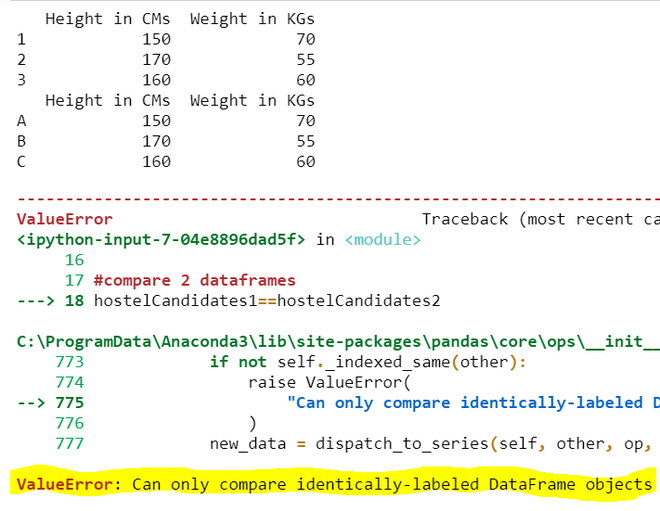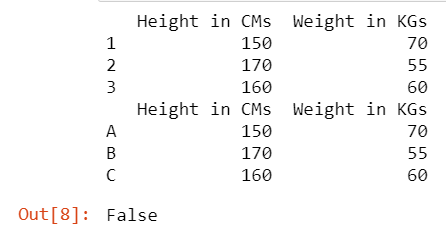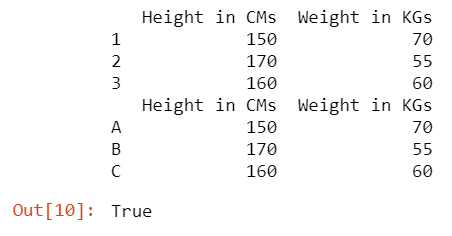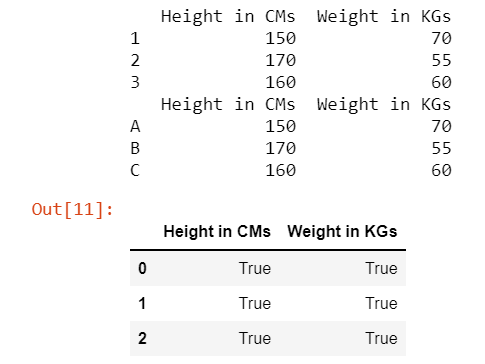# How to Fix: Can only compare identically-labeled series objects

• Last Updated : 12 Dec, 2021

In this article, we are going to see how to fix it: Can only compare identically-labeled series objects in Python.

## Reason for Error

Can only compare identically-labeled series objects: It is Value Error, occurred when we compare 2 different DataFrames (Pandas 2-D Data Structure). If we compare DataFrames which are having different labels or indexes then this error can be thrown.

## Python3

 `# import necessary packages` `import` `pandas as pd`   `# create 2 dataframes with different indexes` `hostelCandidates1 ``=` `pd.DataFrame({``'Height in CMs'``: [``150``, ``170``, ``160``],` `                                  ``'Weight in KGs'``: [``70``, ``55``, ``60``]},` `                                 ``index``=``[``1``, ``2``, ``3``])`   `hostelCandidates2 ``=` `pd.DataFrame({``'Height in CMs'``: [``150``, ``170``, ``160``],` `                                  ``'Weight in KGs'``: [``70``, ``55``, ``60``]},` `                                 ``index``=``[``'A'``, ``'B'``, ``'C'``])`   `# displaying 2 dataframes` `print``(hostelCandidates1)` `print``(hostelCandidates2)`   `# compare 2 dataframes` `hostelCandidates1 ``=``=` `hostelCandidates2`

Output:Even though the data in the 2 DataFrames are the same but the indexes of these are different. So in order to compare the data of 2 DataFrames are the same or not, we need to follow the below approaches/solutions

## Method 1: With consideration of indexes

Here we compare data along with index labels between DataFrames to specify whether they are the same or not. So instead of ‘==’ use equals method while the comparison.

## Python3

 `# import necessary packages` `import` `pandas as pd`   `# create 2 dataframes with different indexes` `hostelCandidates1 ``=` `pd.DataFrame({``'Height in CMs'``: ` `                                  ``[``150``, ``170``, ``160``],` `                                  ``'Weight in KGs'``:` `                                  ``[``70``, ``55``, ``60``]},` `                                 ``index``=``[``1``, ``2``, ``3``])`   `hostelCandidates2 ``=` `pd.DataFrame({``'Height in CMs'``:` `                                  ``[``150``, ``170``, ``160``],` `                                  ``'Weight in KGs'``:` `                                  ``[``70``, ``55``, ``60``]},` `                                 ``index``=``[``'A'``, ``'B'``, ``'C'``])`   `# displaying 2 dataframes` `print``(hostelCandidates1)` `print``(hostelCandidates2)`   `# compare 2 dataframes` `hostelCandidates1.equals(hostelCandidates2)`

Output:As the data is the same but the index labels of these 2 data frames are different so it returns false instead of an error.

## Method 2: Without consideration of indexes

To drop indexes of DataFrame use reset_index method. By dropping the indexes, makes an easy task such that interpreters just check the data irrespective of index values.

Syntax: dataframeName.reset_index(drop=True)

There are 2 ways of comparing data:

• Whole DataFrame
• Row by Row

Example 1: Entire DataFrame Comparison

## Python3

 `# import necessary packages` `import` `pandas as pd`   `# create 2 dataframes with different indexes` `hostelCandidates1 ``=` `pd.DataFrame({``'Height in CMs'``:` `                                  ``[``150``, ``170``, ``160``],` `                                  ``'Weight in KGs'``:` `                                  ``[``70``, ``55``, ``60``]},` `                                 ``index``=``[``1``, ``2``, ``3``])`   `hostelCandidates2 ``=` `pd.DataFrame({``'Height in CMs'``: ` `                                  ``[``150``, ``170``, ``160``],` `                                  ``'Weight in KGs'``: ` `                                  ``[``70``, ``55``, ``60``]},` `                                 ``index``=``[``'A'``, ``'B'``, ``'C'``])`   `# displaying 2 dataframes` `print``(hostelCandidates1)` `print``(hostelCandidates2)`   `# compare 2 dataframes` `hostelCandidates1.reset_index(drop``=``True``).equals(` `    ``hostelCandidates2.reset_index(drop``=``True``))`

Output:Here the data is the same and even though the indexes are different we are comparing the DataFrames by eliminating the index labels so it returns true.

Example 2: Row by Row Comparison

## Python3

 `# import necessary packages` `import` `pandas as pd`   `# create 2 dataframes with different indexes` `hostelCandidates1 ``=` `pd.DataFrame({``'Height in CMs'``: ` `                                  ``[``150``, ``170``, ``160``],` `                                  ``'Weight in KGs'``: ` `                                  ``[``70``, ``55``, ``60``]},` `                                 ``index``=``[``1``, ``2``, ``3``])`   `hostelCandidates2 ``=` `pd.DataFrame({``'Height in CMs'``:` `                                  ``[``150``, ``170``, ``160``],` `                                  ``'Weight in KGs'``: ` `                                  ``[``70``, ``55``, ``60``]},` `                                 ``index``=``[``'A'``, ``'B'``, ``'C'``])`   `# displaying 2 dataframes` `print``(hostelCandidates1)` `print``(hostelCandidates2)`   `# compare 2 dataframes` `hostelCandidates1.reset_index(` `    ``drop``=``True``) ``=``=` `hostelCandidates2.reset_index(drop``=``True``)`

Output:This approach helps us to identify where there are differences between 2 DataFrames and do not compare its index labels as their index labels are dropped while comparison.

My Personal Notes arrow_drop_up
Recommended Articles
Page :